Latest Banking jobs   »   Reasoning Ability Quiz For IBPS Clerk/NIACL...

# Reasoning Ability Quiz For IBPS Clerk/NIACL AO Prelims 2021- 30th September

Directions (1-5): Read the given information carefully and answer the questions;
Seven boxes M, N, O, P, Q, R and S are placed one above another, also they are of different shapes i.e. circle, sphere, cone, triangle, cube, cylinder, square (but not necessarily in the same order).
Only one box is placed between box N and R. Three boxes are placed between box M and the box which is of square shaped but one of them is placed at top. Box P is of triangle shaped. Box S is placed just below box O. Sphere shaped box is placed either at top or bottom. Box P is placed above box O but below box Q. Box R is of square shaped. The box which is placed just below M is of cylinder shaped. Neither Q nor N is of cube shaped box. Cone shaped box is not placed just above box P. Two boxes are placed between box N and box O. Box M is placed below the box which is of the cone shaped.

Q1. What is the shape of Box O?
(a) Circle
(b) Square
(c) Cone
(d) Cylinder
(e) None of these

Q2. Which of the following box is placed at top?
(a) N
(b) S
(c) R
(d) M
(e) None of these

Q3. How many boxes are placed between box Q and box S?
(a) One
(b) Three
(c) Two
(d) More than three
(e) None of these

Q4. Which box is placed just above box N?
(a) S
(b) P
(c) The box which is of cone shaped
(d) Q
(e) Both (c) and (d)

Q5. What is the shape of Box N?
(a) Circle
(b) Square
(c) Cone
(d) Cylinder
(e) None of these

Directions (6-10): Study the following information carefully and answer the questions given below.
In a certain code language,
‘Reading of the Great habit’ is written as ‘doo see mo nee ti’,
‘habit Reading published’ is written as ‘see jo mo’,
‘published by authority’ is written as ‘jo pi ga’,
‘Reading of authority’ is written as ‘pi see ti’.

Q6. Which of the following may represents the code for ‘habit notiﬁcation by authority’?
(a) see ga pi mo
(b) mo pi see nee
(c) ga pi mo ti
(d)jo mo pi ga
(e) yk ga pi mo

Q7. Which of the following could be a code for ‘habit declares authority’?
(a) alu pi ga
(b) alu pi ga
(c) pi ga mo
(d) ga see mo
(e) mo pi kl

Q8. Which of the following is the code for ‘Great’?
(a) nee
(b) doo
(c) mo
(d) either doo or nee
(e) None of these

Q9. What does ‘see’ stand for?
(b) the
(c) Either ‘Great’ or ‘the’
(d) Great
(e) None of these

Q10. What does ‘ga’ stand for?
(a) by
(b) published
(c) authority
(d) Can’t say
(e) None of these

Directions (11-13): Read the following information carefully and answer the following questions.
The distance between point A and B is 3 meters. Point B is 3 meters to the south of point C. Point E is to the east of point A. Points E, A and B are in straight line. C is to the north west of point E. Shortest distance between point C and E is 5 meters. Distance between E and A is more than 1m.

Q11. What is the distance between point A and E?
(a) 7 meters
(b) 6 meters
(c) 11 meters
(d) 9 meters
(e) None of these

Q12. In which direction is point B with respect to point A?
(a) East
(b) West
(c) North
(d) South
(e) None of these

Q13. Five persons viz. A, B, C, D and E have different weights. A is heavier than at least two persons. D is heavier than B. Only C and E are lighter than B. Who among them is the second heaviest?
(a) A
(b) D
(c) B
(d) Cannot be determined
(e) None of these

Q14. In a row of ticket distribution, Arpit stands 11th from starting of the row and Monu is 7th from the end of the row. If there are 5 people standing in between Arpit and Monu then find out how many tickets sold by the vendor (provided each person purchased only one ticket)?
(a) 21
(b) 24
(c) 22
(d) 23
(e) None of these

Q15.In a row A is 8th from the left end and B is sixteenth from the right end of the row if C is 4th from the right of A and fifth from the left of B then find out how many people sit in the row?
(a) 31
(b) 30
(c) 32
(d) 33
(e) None of these

Solutions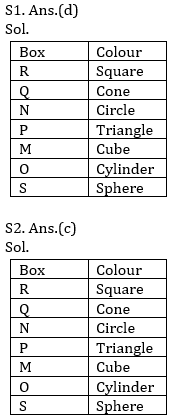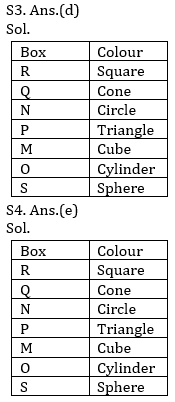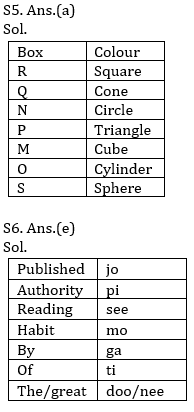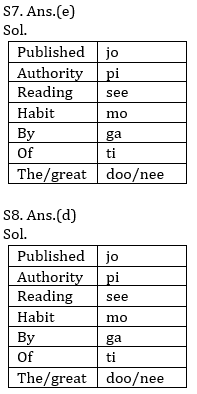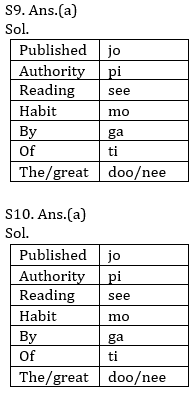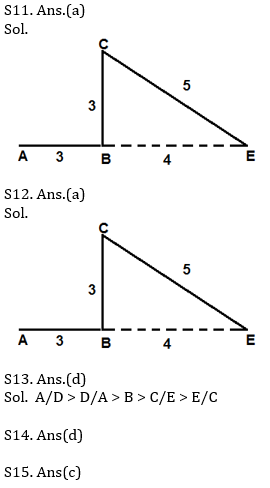Click Here to Register for Bank Exams 2021 Preparation Material#### Congratulations!Download Hindu Review of October 2021: Free PDF